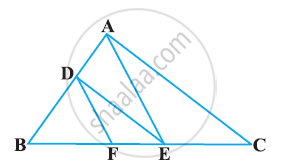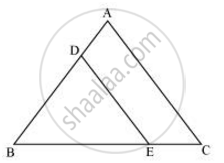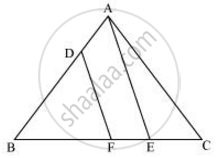# In the following figure, DE || AC and DF || AE. Prove that (BF)/(FE)=(BE)/(EC) - Mathematics

In the following figure, DE || AC and DF || AE. Prove that (BF)/(FE)=(BE)/(EC)#### SolutionIn ΔABC, DE || AC

:.(BD)/(DA)=(BE)/(EC)   (Basic Proportionality Theorem)...(i)In ΔBAE,DF || AE

:. (BD)/(DA) = (BF)/(FE)  (Basic Proportionality Theorem)  ... (ii)

∴ From i and ii we obtain

(BE)/(EC)=(BF)/(FE)

Concept: Similarity of Triangles
Is there an error in this question or solution?

#### APPEARS IN

NCERT Class 10 Maths
Chapter 6 Triangles
Exercise 6.2 | Q 4 | Page 128